Courses
Courses for Kids
Free study material
Free LIVE classes
More

# Division Algorithm FormulaLIVE
Join Vedantu’s FREE Mastercalss

## Introduction

The division algorithm formula is a systematic way of verifying the long division of two numbers or two polynomials. The division formula is a less tedious way of verifying the long division method. Let us learn more about the division algorithm.

### What is the Division algorithm?

Before we dive into using the division algorithm with polynomials we first need to understand what a division algorithm is. Euclid gave the division algorithm. You must be familiar with dividing two numbers. [say] ${\rm{dividend}} = {\rm{divisor}} \times {\rm{quotient}} + {\rm{remainder}}$ This is Euclid's division algorithm. The dividend is the number being divided, the divisor is the number that divides the dividend, and the quotient is the quantity when multiplied by the divisor giving the closest multiple less than the dividend. The remainder is the residue left at the end of the division process. We note that the remainder is always less than the quotient and divisor.Division algorithm

### Division Algorithm Formula for Numbers

The division algorithm formula in numbers states that when a positive number a is divided by another positive number b, we get a unique quotient q and a unique remainder r. Here we say that q and r are positive integers and r is greater than or equal to 0 and less than b.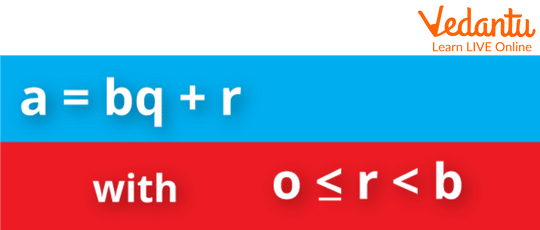Division Algorithm in Numbers

### Properties of Division Algorithm

1. A positive number can only be divided by a smaller number.

2. If the divisor is not a factor of the dividend, then a non-zero remainder will be left behind.

3. There is a unique pair of quotients and remainder.

### Steps to do Division of Two Numbers

1. Always remember that the divisor is always smaller than the dividend

2. First, notice the first digit from the left of the dividend. if the divisor is less than the first digit then take the 2nd digit as well, and put a 0 in the quotient as many times as you find the dividend less than the divisor and keep repeating this process till you get the dividend greater than or equal to the divisor.

3. now write the largest multiple of the divisor that is less than the dividend and write the quotient.

4. Subtract the multiple from the dividend and write down the next digit of the original dividend.

5. Repeat steps 2, 3, and 4. Till you get a remainder smaller than the divisor.

### Solved Examples

1. Divide the numbers 6 and 250.

Ans. Here the divisor is 6 and the dividend is 250.

Step1. First, we see that 6 cannot divide 2 so we write 0 and include the 5 in our dividend.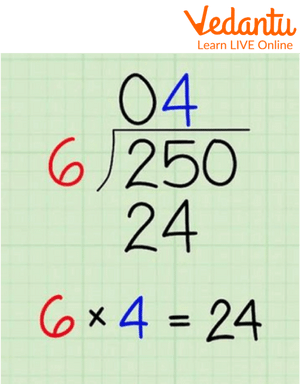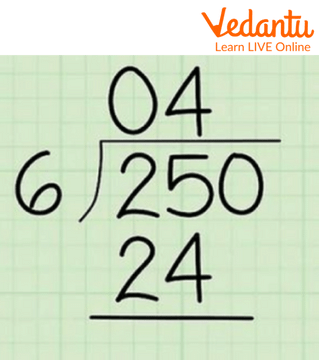Division Algorithm example

Step2. Now we see that 25 is greater than 6 so we start the division process. We will write the largest multiple of 6 which is less than 25. We have 6 x 4 = 24. So 4 will be our quotient and 24 will now be subtracted from the dividend.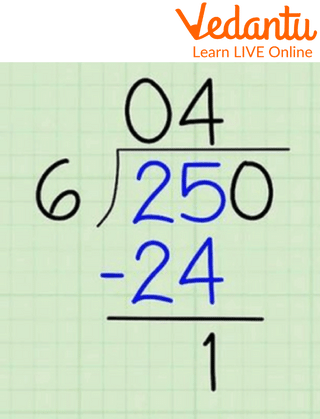Division Algorithm example

Step3. This gives 1 as the remainder. Now we use the 0 left in the dividend. Now our new dividend is 10.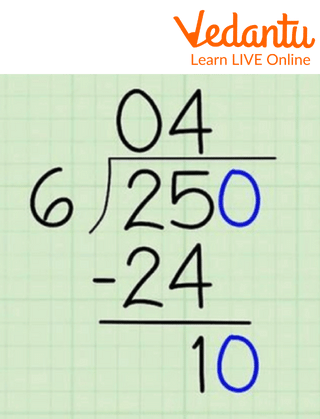Division Algorithm example

Step4. Now we can see 6 x 1 = 6. So 1 will be our quotient and we will have a remainder of 10 - 6 = 4. As we can see 4 is less than 6, and we are finished with the problem. Hence, our quotient is 41 and the remainder is 4 and the division algorithm formula will be, 250 = ( 6 x 41 ) + 4.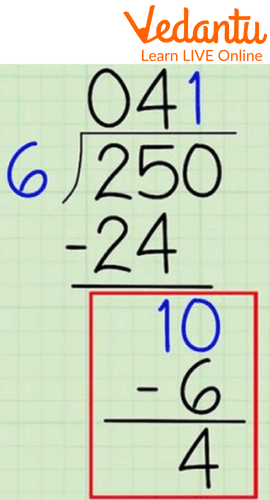Division Algorithm example

2. Divide 813 by 9 and verify the result by the division algorithm.

Ans. We have a divisor as 9 and dividend as 813.

We first start with 9 and 8, we see that 8 is less than 9 so we use 1 as well. Now we see that 9 x 9 = 81. so 9 will be our quotient and we write down 81 under 81 and subtract it. We get 0 as the remainder now we use 3 from the original dividend. Now we see that 3 is less than 9 so we add 0 at the end of the quotient, so our quotient is 90, and we have a remainder of 3. Now we need to verify this, we have

813 = ( 9 x 90 ) + 3 from the division algorithm formula

813 = 810 + 3

813 = 813

Hence, verified.

### Conclusion

A division algorithm is an essential tool for verifying and proving important facts in mathematics. We have learned how to use the division algorithm formula. We have learned some of its important properties and solved several examples.

Last updated date: 29th Sep 2023
Total views: 82.8k
Views today: 2.82k

## FAQs on Division Algorithm Formula

1. Who gave the division algorithm formula?

Euclid gave the division algorithm formula.

2. What is the condition of the remainder in the division algorithm?

For any pair of divisor and dividend, the remainder should be greater than or equal to 0 and always less than the divisor.

3. Why does the division algorithm work?

The division algorithm is a compact way of writing long division. We use it to check and verify the correctness of the long division of two numbers.

4. Is it possible to divide two negative numbers? what will be the quotient when we divide two negative numbers?

Yes, we can divide two negative numbers. The division process proceeds the same as for positive numbers. When we divide two negative numbers we get a positive number in the quotient.

5. What will be the quotient when we divide a negative and a positive number?

When we divide a positive and a negative number, the division process is always the same as we learned. Except that the quotient will be a negative number regardless if the divisor is positive and the dividend is negative or if the divisor is negative and the dividend is positive.